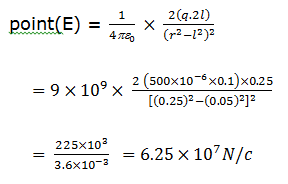# The electric intensity due to a dipole of length 10 cm and having a charge of 500 mC, at a point on the axis at a distance 20 cm from one of the charges in air, is         (a)    6.25 × 107 N/C (b)    9.28 × 107 N/C (c)    13.1 × 1011 N/C (d)    20.5 × 107 N/CAditi Chauhan
9 years ago

(a)

Given: Length of the dipole (2) = 10cm = 0.1 m or  = 0.05 m

Charge on the dipole (q) = 500 pC = 500 x 10-6 C and distance of the point on the axis from the mid-point of the dipole (r) = 20 + 5 = 25 cm = 0.25 m.

We know that the electric field intensity due to dipole on the given2 years ago
Dear Student,

Length if dipole = 10cm
Distance from point of axis = 20cm
Charge = 500 μC
The electric field intensity due a dipole at a point on the axial line is given as -
E = 2kpr/(r²-l²)2
where p is the dipole moment of dipole, r is the separation between midpoint of dipole to the observation point.
500 μC on each pole of dipole and separation between two dipoles will be 2l = 10cm
Dipole moment , p = 500 × 100cm
= 500 × 10^-6 C × 0.1 m
= 5 × 10^-5 C.m
Distance between the observation point to one charge, d = 20cm
Distance between observation points to midpoint of dipole moment , r = (d + x/2) = 20cm + 5cm = 25cm = 0.25m
E = 2 × 9 × 10^9 × 5 × 10^-5 × 0.25/(0.25² - 0.05²)²
= 90 × 10⁴ × 0.25/(0.30)²(0.20)²
= 2.25 × 10^5/(0.09 × 0.04)
= 2.25/(36) × 10^9
= 225/36 × 10^7
= 6.25 × 10^7 N/C
Thus, one of the charges in air, is 6.25 × 10^7 N/C.

Thanks and Regards